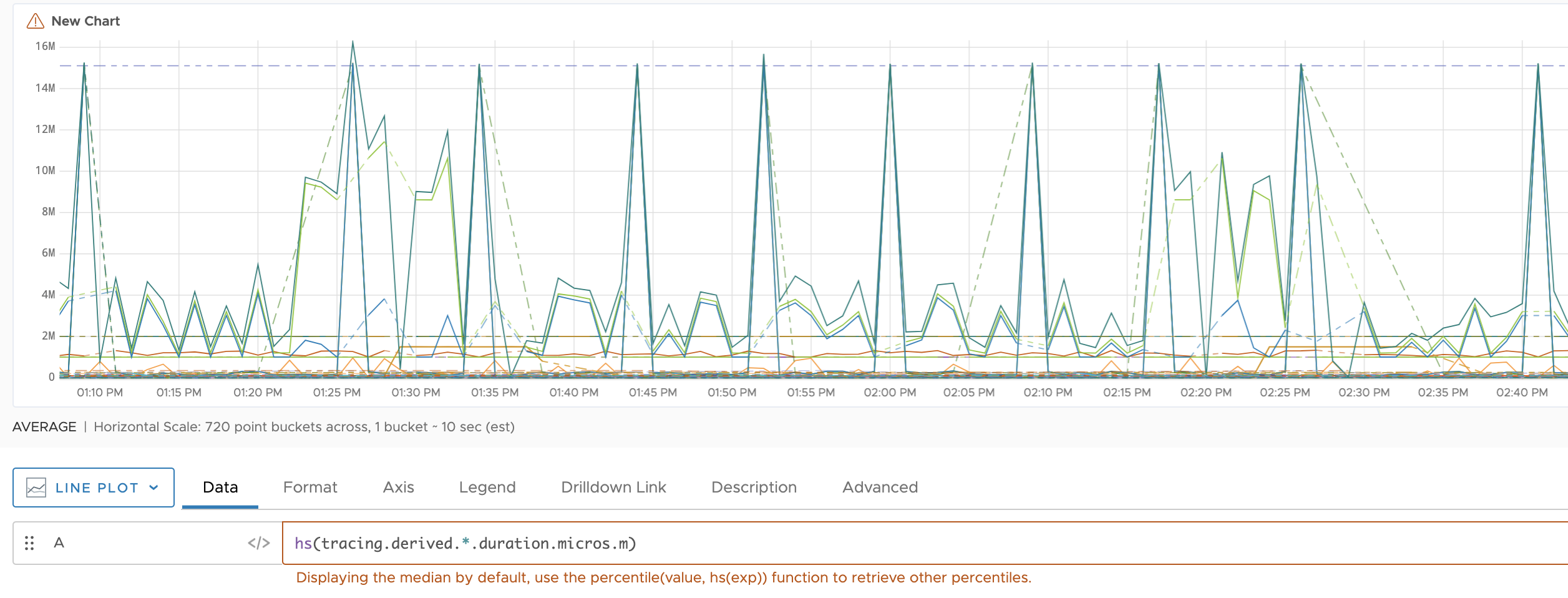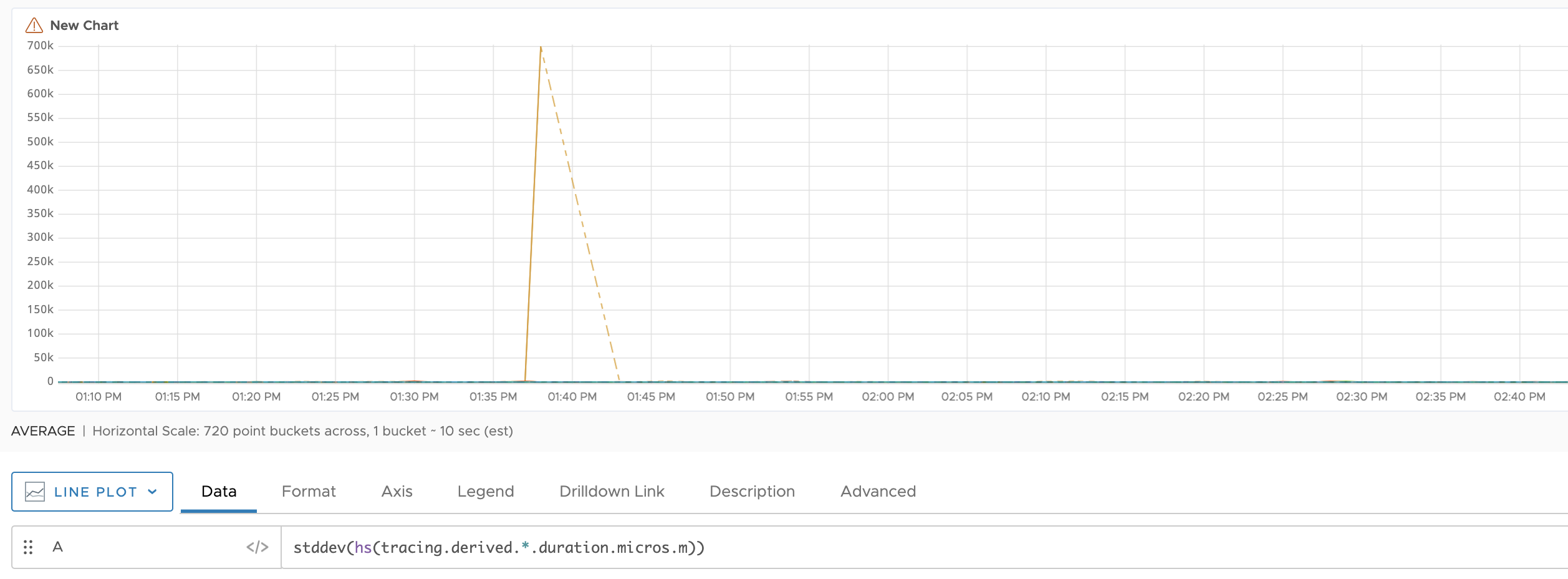Reference to the stddev() function

## Summary

``````stddev(<hsExpression>)
``````

Shows how the data in a histogram expression is distributed around the mean.

## Parameters

ParameterDescription
hsExpression Expression describing the histogram series.

## Description

The `stddev()` function shows you how the data in a histogram expression varies against the mean or average and returns those values as a time series. A separate time series is returned for each histogram series. You can use this function to understand the volatility of your data or to find anomalies in your histogram expression.

## Example

This chart represents all the histogram series described by `hs(tracing.derived.*.duration.micros.m))`. Each histogram series consists of distributions from a particular source, and a given source might emit more than one histogram series. The chart represents each histogram series as a separate line that shows the median values of the distributions.Use the following query to find the standard deviation:

``````stddev(hs(tracing.derived.*.duration.micros.m)))
``````

Now, you see how the data in your expression varies against the mean. You see an anomaly (a spike) between 1.35 PM and 1.45 AM.